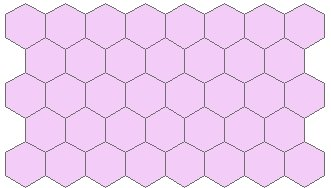Go to ET GeoWizards 12.x User Guide

Create Tiles

Go to ToolBox Implementation

Creates a regular polygons grid with user defined extents, tile shape and size.

Inputs:

• Initial extent of the grid - the grid will be generated using the coordinate system and units of:
• the current view
• a layer from the current view
• Output spatial reference - the grid will be stored in the spatial reference of
• the current view
• a layer from the current view
• Grid type
• Triangle
• Square
• Hexagon
• Output spatial reference
• Cell size.
• Size represents option - depending on the user input the size parameter can represent
• The side of the polygon
• The radius of the circle inscribed in the polygon
• The radius of the circle circumscribed around the polygon.
• Shape orientation (for square and hexagon tiles only) - see examples below
• Flat
• Pointy

Outputs:

• New Polygon feature class

Notes:

• The initial extents of the grid are defined by the extents of the selected layer or current extents of the data frame. The extents can be changed manually during the dialog.
• The cell size will be in the units of the input layer or data frame.
• If the input is Unprojected only values between X = -180 To 180 and Y = -90 To 90 will be accepted
• If the Input is Projected and the Output is in different projection, the user should make sure that the Grid extents are valid
• In order to avoid incorrect inputs, the size of the grid is limited to 4,000,000 cells (2000 by 2000)
• A ET_Index field will be added to the attribute table. The values will indicate the index of each Grid cell. The cell in the bottom left corner of the Grid will have an index of "0-0".

Examples:

 Shape = TriangleShape = Square, Orientation = "Pointy"Shape = Hexagon, Orientation = "Flat"Shape = Hexagon, Orientation = "Pointy"Command line syntax - ET_GPCreateTilesExtent

ET_GPCreateTilesExtent <out_feature_class> {out_spatial_reference} <shape_type> {get_extents_from} <Extent> <Size_represents> <Size> {Orientation}

Parameters

Expression Explanation
<out_feature class> A String - the full name of the output feature class (A feature class with the same full name should not exist)
{out_spatial_reference} The spatial reference of the output feature class. If not specified the output will be created with Unknown coordinate system
<shape_type> A String controlling the shape type of the output tiles - "Triangle", "Square" or "Hexagon"
{get_extents_from} A String - a layer name or the full name of the a feature class to be used as a source for the extents.
<Extent> An Envelope representing the extents - "XMin YMin XMax YMax"
<Size_represents> Required. A String indicating the meaning of the <SIZE> parameter
• Side - the side of the polygon
<Size> A Double representing the size (see above for options)
{Orientation} A String representing the tile orientation - "Flat" or "Pointy" - for Square or Hexagon tiles only.

Scripting syntax - ET_GPCreateTilesExtent

ET_GPCreateTilesExtent (out_feature_ class, out_spatial_reference, shape_type, get_extents_from, Extent,  Size_represents, Size, Orientation)

Command line syntax - ET_GPCreateTilesOrigin

ET_GPCreateTilesOrigin <out_feature_class> {out_spatial_reference} <shape_type> <X_origin> <Y_origin> <Number_columns> <Number_rows> <Size_represents> <Size> {Orientation}

Parameters

Expression Explanation
<out_feature class> A String - the full name of the output feature class (A feature class with the same full name should not exist)
{out_spatial_reference} The spatial reference of the output feature class. If not specified the output will be created with Unknown coordinate system
<shape_type> A String controlling the shape type of the output tiles - "Triangle", "Square" or "Hexagon"
<X_origin> A Double representing the X coordinate of the lower left corner of the grid.
<Y_origin> A Double representing the Y coordinate of the lower left corner of the grid.
<Number_Columns> A Long representing the number of columns that the resulting grid will have
<Number_Rows> A Long representing the number of rows  that the resulting grid will have
<Size_represents> Required. A String indicating the meaning of the <SIZE> parameter
• Side - the side of the polygon
<Size> A Double representing the size (see above for options)
{Orientation} A String representing the tile orientation - "Flat" or "Pointy" - for Square or Hexagon tiles only.

Scripting syntax - ET_GPCreateTilesOrigin

ET_GPCreateTilesOrigin (out_feature_ class, out_spatial_reference, shape_type, X_origin, Y_origin, Number_Columns, Number_Rows, Size_represents, Size, Orientation)

See the explanations above:
<> - required parameter
{} - optional parameter